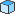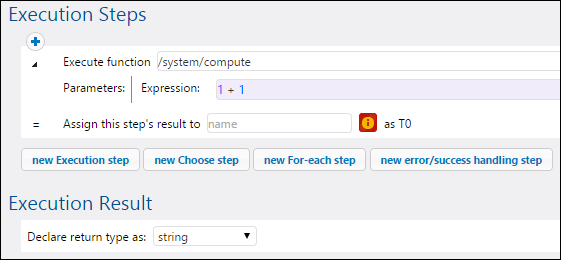# Altova FlowForce Server 2023 Advanced Edition

## Compute an Expression

A simple way to test FlowForce expressions before embedding them in jobs is to create an execution step that calls the/system/compute function. For a step-by-step example, see Creating a "Hello, World!" Job.

The/system/compute function evaluates the value of the Expression parameter and returns the computed result. Importantly, this function has no defined return type. The actual type depends on the expression being computed. For example, if you pass to this function the expression 1+1, the function returns the numeric value 2. However, if you pass to this function the expression '1+1', it returns the string value 1+1.

To understand this concept better, create a step that calls the/system/compute function and enter "1+1" in the expression field. Make sure to declare the job return type as "string", as shown below.When you attempt to save the job, FlowForce displays a "Types string and number do not match" error. This error happens because the computed expression is a number, whereas the return type of the job is declared as a string value.

To fix the typing problem, either change the return type of the job to "number" or convert the number to a string. The example below calls the FlowForce expression function string which converts a number into a string value.When you need to compute an expression and return the value as string, you can alternatively use the/system/compute-string function. In this case, note that the expression part must be delimited from the string with curly braces (see Embedding Expressions in String Fields ).